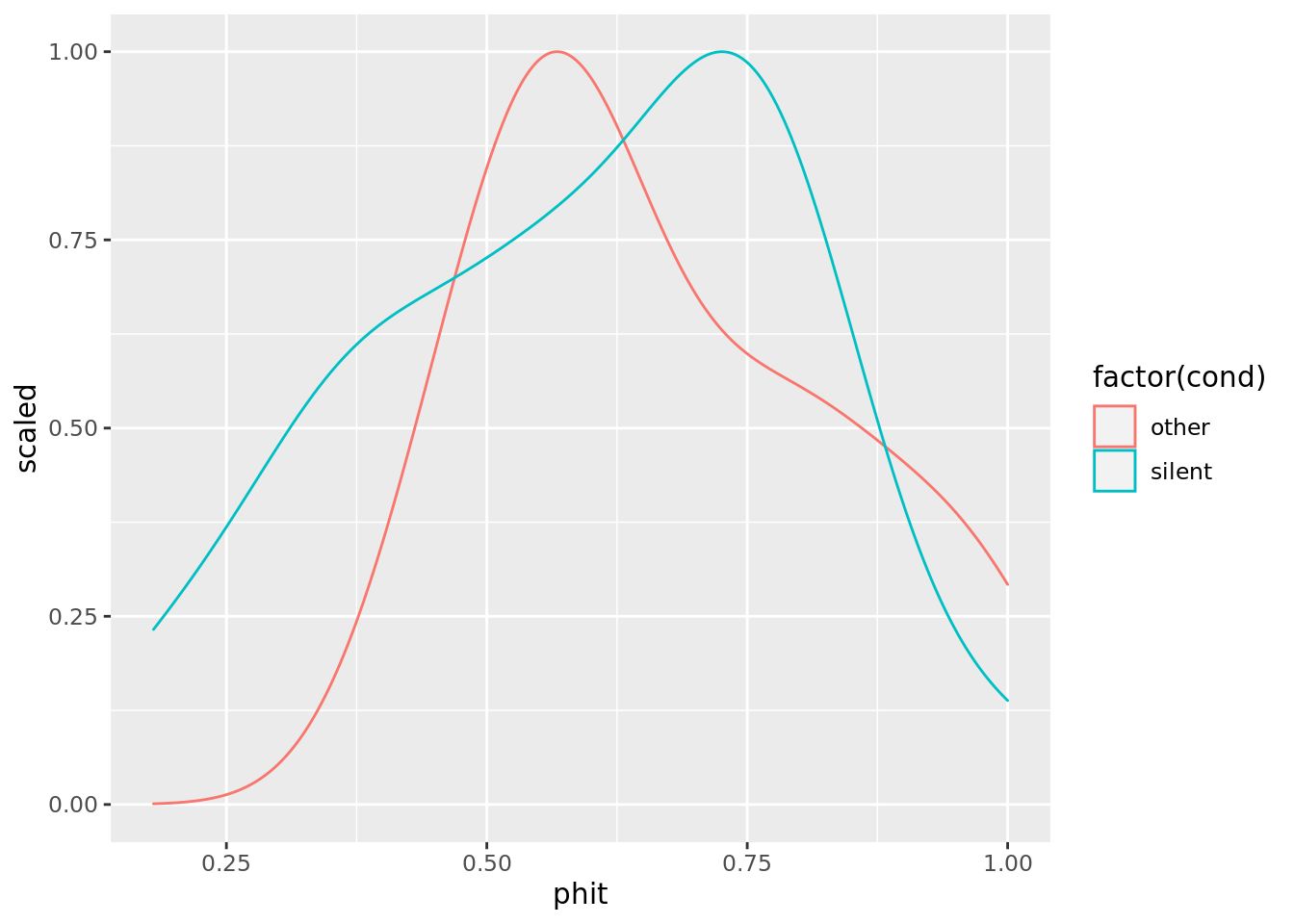In this worksheet, you’ll revise concepts you’ve already learned. We’ll use these concepts in the next worksheet.

In this worksheet, we’ll analyse some data on the production effect in memory. In this particular production-effect experiment, the experimenters examined whether people remember words better if they read them silently, or if someone else reads the words out loud to them. It was a between-subjects experiment; in other words, some participants read silently, while others had the words read out loud to them. All participants were later given a recognition test, where they had to pick out the presented words from a list that contained both old and new words. These data were computer-generated for the purposes of this exercise, but are similar to real data in this area.

First, you need to get the data and load it into Rstudio. Here’s how:

• Start a new RStudio project and name it (Plymouth University students: use the name psyc520).

• Open an R script ready to record all your commands, and save it as revision.R

• Use RStudio to upload the production effect data to that project.

• Load the data into a dataframe.

Now, take a look at the data by clicking on its name in the Environment tab. You will see there are three columns, named subj, cond, and phit. The subj column is an anonymous ID number for each participant. The cond column indicates which of the two conditions of the experiment the participant was in. The phit column gives the probability of a ‘hit’. This is a measure of how good that participant’s memory was (bigger is better).

## Descriptive statistics

Next, take a look at how much the groups (silent vs. other) differ from each other, and how much variability there is in each group. Specifically:

# A tibble: 2 x 2
cond   mean(phit)
<chr>         <dbl>
1 other         0.671
2 silent        0.592
• Look at the variability in each group, and group overlap, using a density plot. It should look like this:## Effect size

In you have not previously been taught about effect sizes, take a look at the materials here before proceeding.

In those materials, you learned that effect size measures how big the difference between two groups is, relative to the amount of variability within each group. For example, you worked out that the gender pay gap in the U.S. is several thousand dollars a year, and that the effect size is small, because both men and women vary a lot in how much they earn. The variability of incomes in the U.S. is high because there are a wide range of incomes above and below the mean.

Calculate the effect size for the current memory experiment. If you get it right, your output should look like this:


Cohen's d

d estimate: 0.4179971 (small)
95 percent confidence interval:
lower      upper
-0.1044576  0.9404518 

Effect size measures how big the difference between the groups is, relative to the amount of variability in each group. Specifically, effect size is the difference in the means, divided by the standard deviation (a measure of variability). An effect size of 0.5 occurs when the difference in the means is half the standard deviation. Anything below 0.5 is considered to be a small effect size. Anything above 0.8 is considered large. Between these two figures, effect sizes are described as ‘medium’.

This experiment has a small effect size.

## Bayes Factor

Bayes Factors measure the level of evidence for there being (or not being) a difference between groups. If you get a Bayes Factor of 3 or more, psychologists generally consider that meaningful evidence for a difference. If you get a Bayes Factor of 0.33 or less, we generally consider that meaningful evidence for the absence of a difference. Where a Bayes Factor is greater than 0.33 but smaller than 3, we generally conclude the experiment didn’t tell us anything worth listening to.

Calculate the Bayes Factor for this experiment. The correct answer is:

Bayes factor analysis
--------------
 Alt., r=0.707 : 0.7811737 ±0.01%

Against denominator:
Null, mu1-mu2 = 0
---
Bayes factor type: BFindepSample, JZS

This experiment didn’t tell us anything worth listening to: the Bayes Factor was close to 1.

## All done!

That’s the end of the revision. You are now ready to start the intermediate-level guide to R.